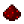# bit.borFunction bit.bor Computes the bitwise inclusive OR of two numbers Syntax bit.bor(number m, number n) Returns number the value of m OR n Part of ComputerCraft API bit

## ExamplesExample OR the number 18 (10010) with the number 3 (00011), yielding 19 (10011) Code ```print(bit.bor(18, 3)) ``` Output 19

## Explanation

All bit operations operate in binary numeral system . An inclusive OR operation between two bits yields a 1 if either of the bits is 1 and a 0 if they are both 0. This function produces an output by computing the OR of each bit of its two inputs independently. So, for the example above:

 Bit index: Input 1 (18): Input 2 (3): Calculation: Output (19): 4 3 2 1 0 1 0 0 1 0 0 0 0 1 1 18 has a 1 Both 0 Both 0 Both 1 3 has a 1 1 0 0 1 1